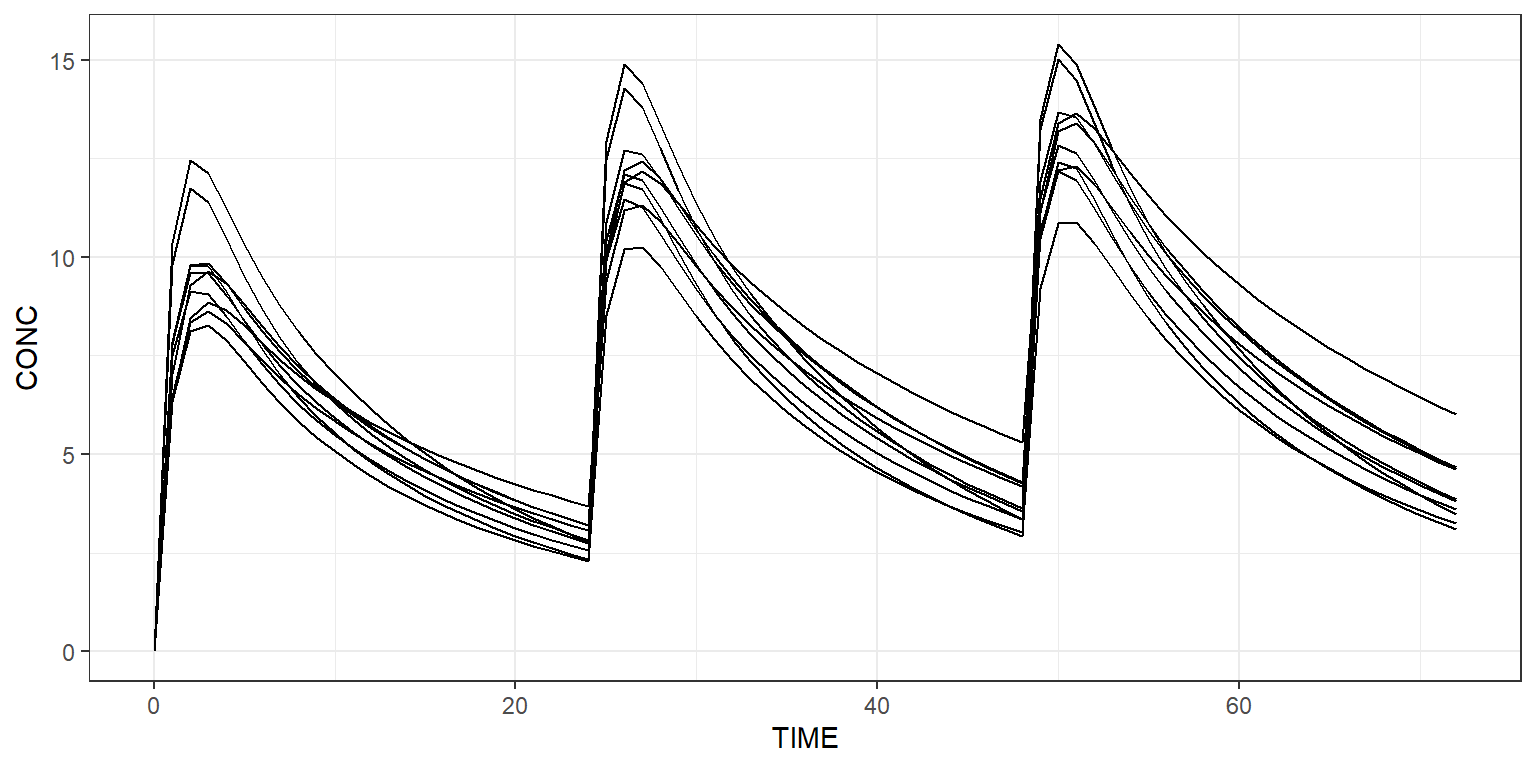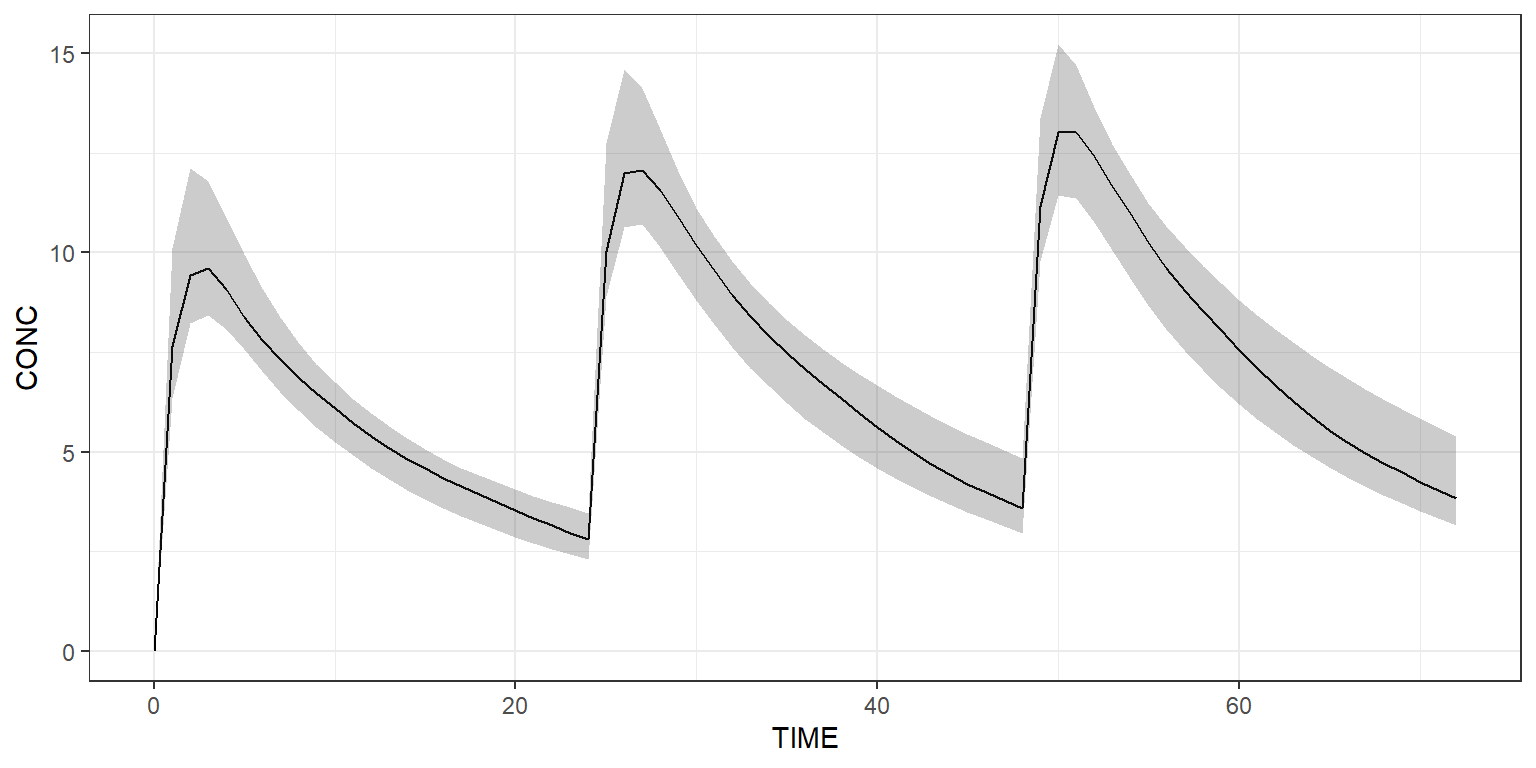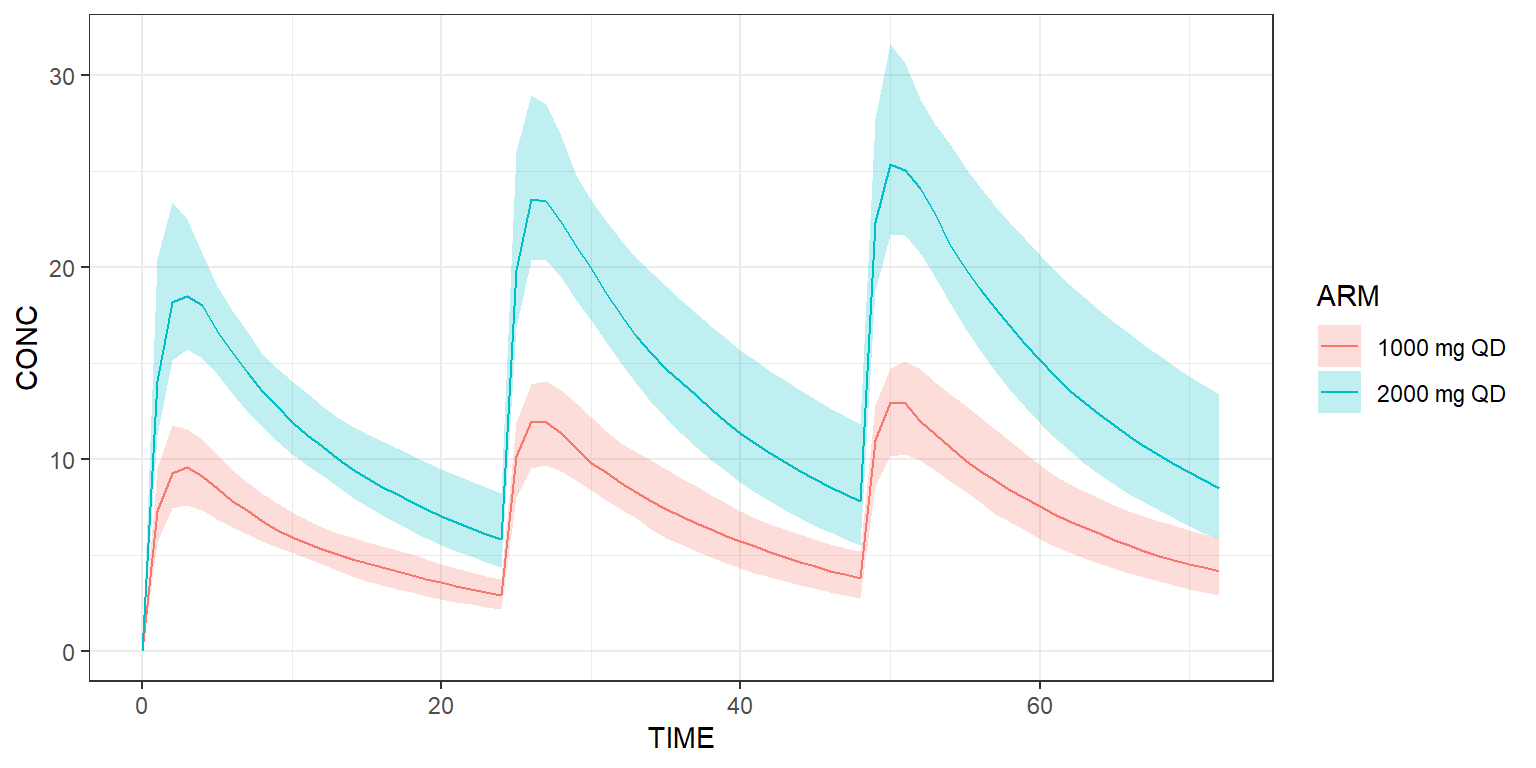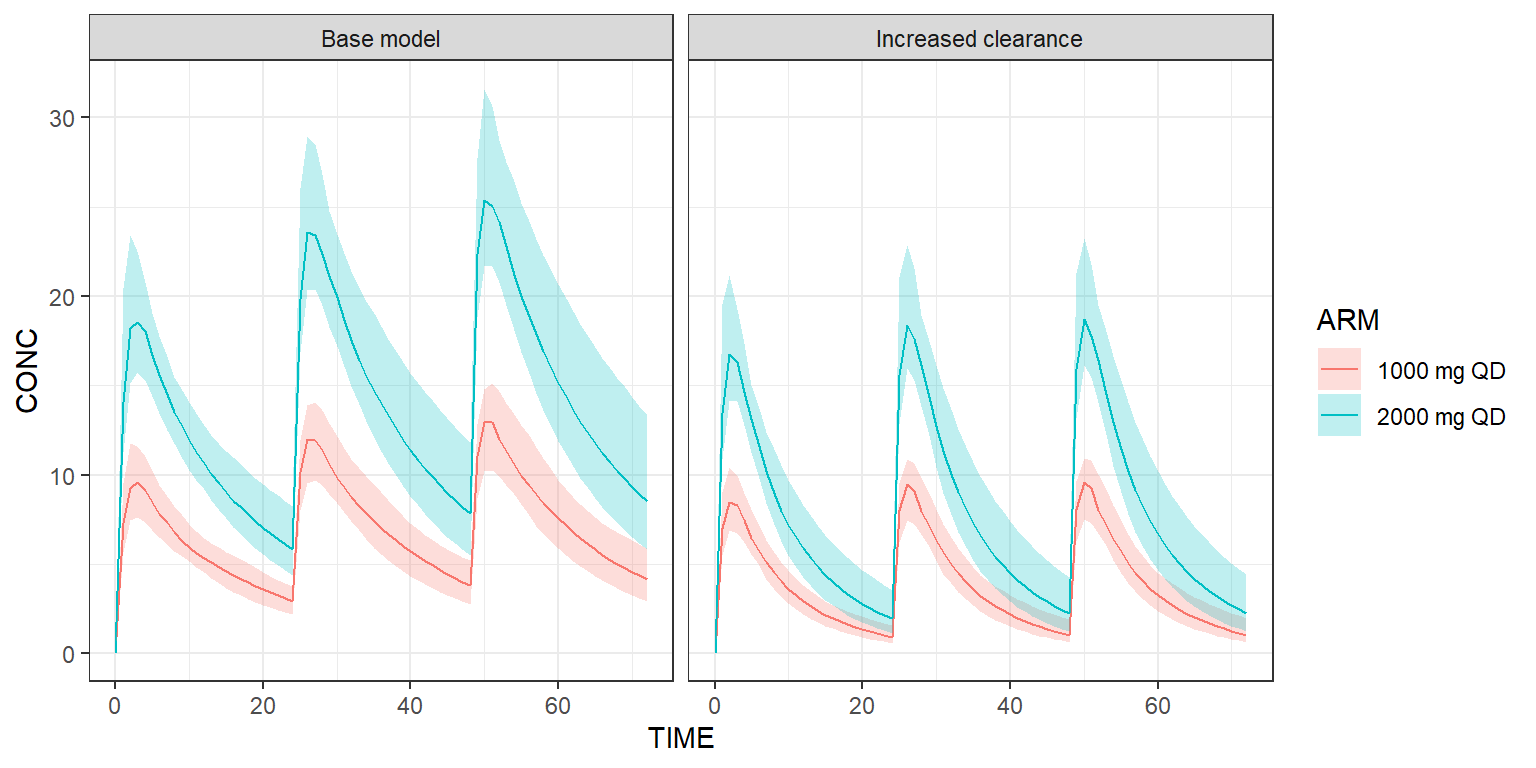library(campsis)

Load a PK model from the built-in model library or load your own one.

model <- model_library\$advan4_trans4 # 2-compartment PK model with absorption compartment

## Create dataset

Create a dataset of 10 individuals in CAMPSIS. For instance, let’s give 1000 mg QD for 3 days and observe every hour.

dataset <- Dataset(10) %>%
add(Observations(times=0:72))

## Simulate

Simulate this very simple protocol:

## Plot results

Plot all simulated profiles using a spaghetti plot:

spaghettiPlot(results, "CP")Or use a shaded plot to see the simulated 90% prediction interval:

shadedPlot(results, "CP")## Simulate arms

The dataset can contain more than one treatment arm. In the example below, we explicitly create two arms. The first arm receives a dose of 1000 mg QD, while the second arm receives twice this dose amount.

arm1 <- Arm(subjects=50, label="1000 mg QD") %>%

arm2 <- Arm(subjects=50, label="2000 mg QD") %>%

dataset <- Dataset() %>%

results <- model %>% simulate(dataset, seed=1)
shadedPlot(results, "CP", scenarios="ARM")## Derive from base model

Scenarios derived from the base model and/or dataset can be easily implemented. Below, we’d like to see what happens if the clearance of this model is multiplied by two.

scenarios <- Scenarios() %>%
add(Scenario("Base model")) %>% # Original CL is 5
add(Scenario("Increased clearance", model=~.x %>% replace(Theta(name="CL", value=10))))

results <- model %>% simulate(dataset, scenarios=scenarios, seed=1)
shadedPlot(results, "CP", scenarios=c("ARM", "SCENARIO")) + ggplot2::facet_wrap(~SCENARIO)